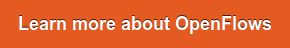# Tweet

I think we all agree that we want pumps to run efficiently. It’s one of the few things in this world that pretty much everyone agrees on. And with advances in SCADA and digital twins, we are doing a much better job collecting data on pump performance. But what is the best metric for judging pump efficiency?

## The Metrics

Wire-to-Water Efficiency

Of course, you can measure the wire-to -water efficiency which is defined as

e = water power/input power = k Q h/P                                                                             (1)

where e = efficiency (as a fraction), Q = flow, h = pump head, P = input power, k = unit conversion factor. For this blog, k will be whatever unit conversion factor is needed for that situation. It will vary depending on the equation and the units in the raw data.

If you know Q, h and P, this is a trivial equation. You just need to get the units right.

Specific Energy

Another metric for efficiency is specific energy. It has multiple definitions in the literature, but the consensus seems to be

S = kP/Qh                                                                                                                                    (2)

Where S = specific energy which has units of power per flow-head.

If you combine equations (1) and (2), you get

S = k/e                                                                                                                                          (3)

So specific energy, if you cancel the units, is just the inverse of efficiency. It is one if the pump is perfectly efficient and increases as the pump becomes less efficient.

Both metrics are correct and useful. Which is better?

My opinion? Efficiency is slightly better. Why?

1. Efficiency is dimensionless which means that an efficiency of 0.8 (80%) means the same thing in Detroit or Dubrovnik. With specific energy, how do you compare a value of 200 kw/(L/s – m) with 11,000 BTU/(gpm – ft). To make any comparison, each calculation needs to be based on the same units. This works if you are comparing energy efficiency in one water utility where everything is done in the same units, but trying to benchmark across multiple systems is tedious and confusing. Plus, everyone intuitively understands what an efficiency of 0.8 means.

1. There already is a concept of “specific energy” in hydraulics which has been in use much longer than specific energy in pumping. It refers to whether the energy in open channel flow is primarily in depth or velocity head. Sure, you can tell the difference between the two specific energies based on context, but why reuse the same term twice?

## Instantaneous vs. Cumulative

The equations above calculate the efficiency at a specific point in time based on the current flow and power input. Efficiency varies over time and understanding this variation can be helpful for operators. There may be certain conditions, especially for variable speed pumps, where pump efficiency can deteriorate significantly in specific situations. Having a trace of efficiency over time can be very useful in getting to the root of the problem.

However, for general management purposes, only the average efficiency may be of interest. In addition, in many cases, the only meters are totalizing meters which give the cumulative energy consumption and

cumulative flow. In this case, equation (1) becomes

e = k V h/E                                                                                                                            (4)

Where V is the volume pumped in which E is the energy used over the same time period.

## Pump vs. Pump Station

In many cases, the power, flow, or both are only measured at the pump station level. That is one flow meter and one power meter per station. While this is not as useful as measuring the efficiency at each pump, the equations above are still valid and they can provide some insights. For example, if the efficiency deteriorates at certain times, checking the pump or pump combination at that time can provide an indication of a problem pump or an undesirable combination.

## Alternative Definitions

There are a variety of different definitions of specific energy. One that troubles me is sometimes referred to as energy intensity

S2 = kP/Q

Where S2 is the alternative specific energy.

The problem here is the need to make some reasonable estimate of the typical pump head. If the pump head is constant, then this is reasonable, but if it varies, there is no way to correct for this variable. Since pump head varies from one pump station to another, even within a single system, it is only useful in calculating the specific energy for a single pump or a battery of several identical pumps.

## Summary

As more and more analytics are being calculated in digital twins, it is important to understand what is being calculated. As far as what is the best metric? Efficiency is my preference.If you like blogs like this, you can go back to our library of blogs at https://blog.virtuosity.com/tag/water-and-wastewater.